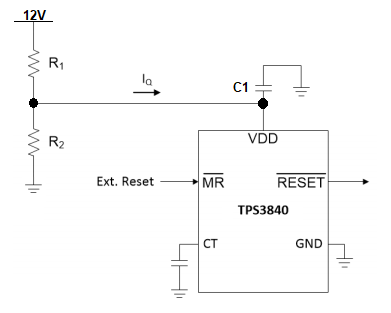If you have a related question, please click the "Ask a related question" button in the top right corner. The newly created question will be automatically linked to this question.

# [FAQ] TPS3840: resistor divider for sensing wide input voltages

Part Number: TPS3840

I need to monitor a 12V rail with 7.6V as the UV threshold. Can I use TPS3840 for this purpose? What precautions do I need to take?”

• Hi Ajay,

Yes, you can use the TPS3840 for this purpose.  The TPS3840 variant that I would suggest is TPS3840DL31DBVR which has a 3.1V threshold voltage.  Because the 12V rail is higher than the absolute maximum for the TPS3840 input voltage (VDD) we would need to use a resistor divider to reduce the voltage at the input (see below).

If we assume a resistor R2 to be 10Kohms, then we can solve for R1 knowing that the UV threshold is 3.1V at the rail voltage of 7.6V.  See below on how to solve for R1:

7.6V * (10K/(R1 + 10K)) = 3.1V

R1 = 14.5Kohms

A standard 1% resistor close in value to 14.5Kohms is 14.7Kohms.  Although the resistor divider may be a viable solution for monitoring higher voltage rails, please keep in mind that using external resistors may introduce voltage monitoring inaccuracies because the resistor themselves are within 1% accurate.  Also, as seen with resistor R1, 14.5Kohms is not a standard 1% resistor value and selecting values not 14.5Kohms will introduce accuracy errors.

Also, always check to ensure that input voltage stays below the recommend operating voltage which is 10V for the TPS3840.

One thing to note, during start-up the TPS3840 will have a slight in-rush current that causes a voltage drop across R1.  The in-rush current usually occurs for tens of microseconds.  Depending on the size of R1, too large of a resistor may cause a large voltage drop at the input of VDD.  If the voltage drop causes the input of VDD to drop below the VPOR spec (950mV for open-drain output option) the TPS3840 will turn off and not allow the output (/RESET) to deassert.  It is suggested that R1 should not be larger than 20Kohms and when using a resistor divider a 1uF capacitor (C1) should be placed at the VDD input to reduce the voltage drop seen at VDD.Ben